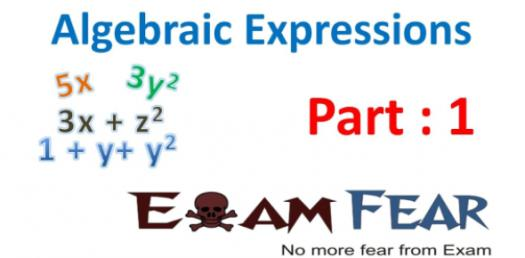# Algebraic Expression Practice Quiz

5 Questions | Total Attempts: 82SettingsWhen it comes to solving an expression one has to ensure that they have set the equation correctly from the narrations given. Do you know how to formulate these expressions correctly? Take up the quiz below and polish your understanding on these problems. Give it a try and all the best!

• 1.
Look at the rectangle below. Which of these expressions represents the perimeter of the rectangle?
• A.

5x – 2(3 + x)

• B.

(5x – 2)(3 + x)

• C.

(5x – 2) + (3 + x)

• D.

2(5x – 2) + 2(3 + x)

• 2.
The school faculty is planning a retirement dinner in honor of three retiring faculty members. The caterer charges an \$80 set-up fee plus \$14 per person. The expression for the total cost is 80 + 14x, where x is the number of people attending. Which of these expressions represents the total cost per person?
• A.
• B.

X(80 + 14x)

• C.
• D.

80x + 14x²

• 3.
A gas and electric bill totals \$324.86. If x represents the charge for electricity, which of these expressions represents the charge for gas?
• A.

\$324.86x

• B.

\$324.86 + x

• C.

\$324.86 – x

• D.

\$324.86 ÷ x

• 4.
The expression 16x² + 28x – 36 represents the perimeter of a square. Which of these expressions represents the length of one side?
• A.
• B.
• C.
• D.
• 5.
Martha is x years old. Esteban is x + 7 years old. Martha's mother is 3x + 5 years old. Which of these expressions represents how much older Martha's mother is than Esteban?
• A.

(x + 7) – x

• B.

(3x + 5) – x

• C.

(x + 7) – (3x + 5)

• D.

(3x + 5) – (x + 7)

Related TopicsBack to top